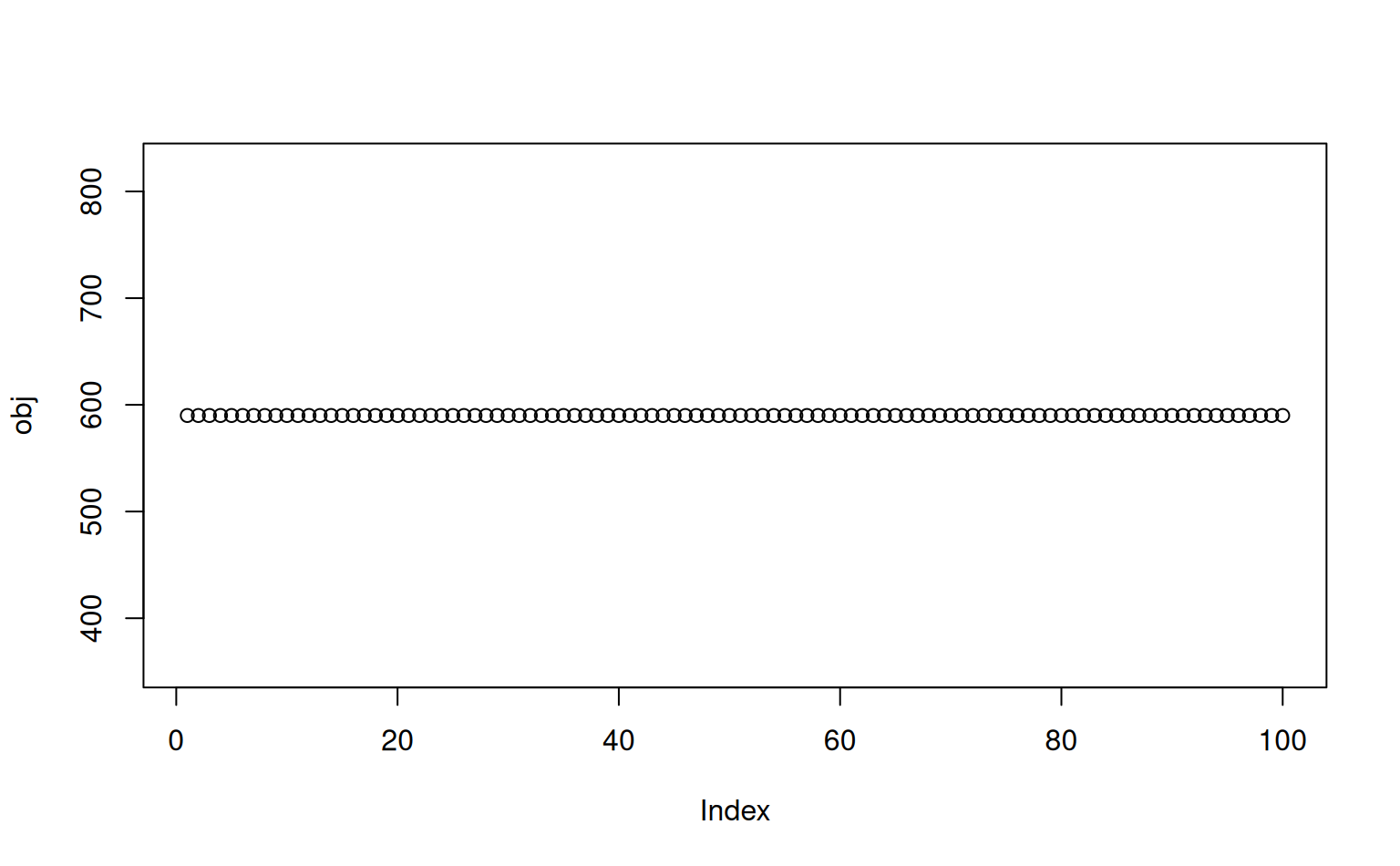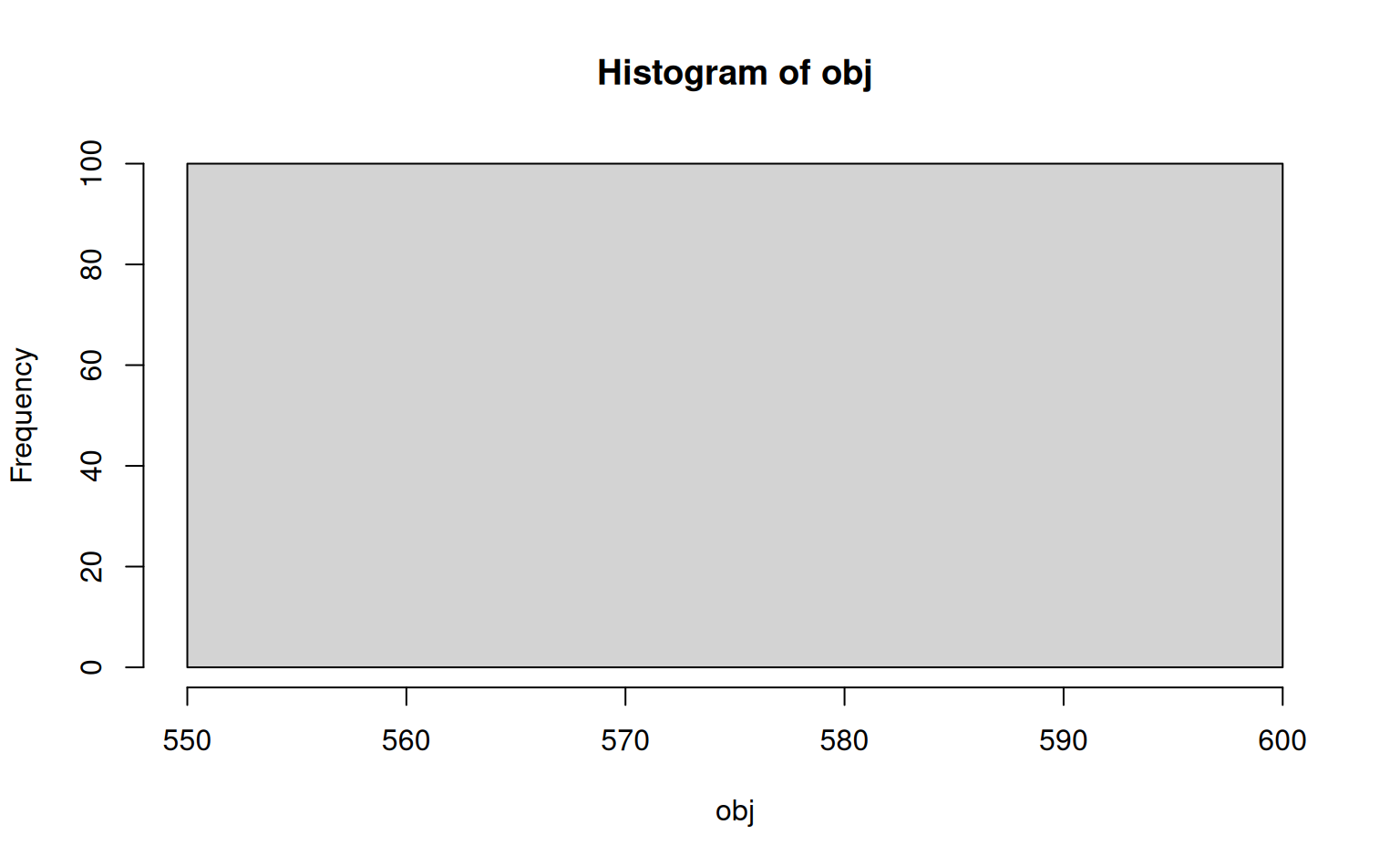Iteratively Attempt Hydrologic Ordering of Geomorphic Proportion Matrix

iterateHydOrder(
x,
g,
target = 0.9,
maxIter = 20,
j.amount = 0.05,
verbose = FALSE,
trace = FALSE
)

## Arguments

x

data.frame geomorphic proportion matrix, as created by soilDB::fetchOSD(..., extended=TRUE)

g

name of geomorphic summary table, one of: c('geomcomp', 'hillpos', 'flats', 'terrace', 'mtnpos', 'shape')

target

numeric, target match rate

maxIter

integer, maximum number of perturbations of geomorphic probability matrix

j.amount

numeric, amount of noise applied to rows with too few unique values, passed to jitter()

verbose

logical, additional output printed via message

trace

logical, additional list of results for each iteration

## Value

A list with the following elements:

• clust: rotated hclust object

• hyd.order: vector of series names, in hydrologic ordering

• clust.hyd.order: vector of series names, after clustering + rotation, approximate hydrologic ordering

• match.rate: fraction of series matching target hydrologic ordering, after clustering + rotation

• obj: objective function value (sum of squared rank differences), used by iterateHydOrder()

• niter: number of iterations

• trace: list of results by iteration, only when trace = TRUE

## Details

This function is used by the suite of geomorphic proportion visualization functions (viz*) to attempt rotation of a dendrogram according to "hydrologic ordering" rules. A perfect rotation is not always possible, and reported as a match rate in the returned score value

D.E. Beaudette

## Examples


# example data, similar to results from soilDB::fetchOSD(..., extended = TRUE)
data("OSDexamples")

# single iteration of hydrologic ordering
h1 <- hydOrder(OSDexamples$hillpos, g = 'hillpos', clust = TRUE) # perform several iterations, keep the best one h2 <- iterateHydOrder(OSDexamples$hillpos, 'hillpos', verbose = TRUE)
#> 17.6% match rate after 20 iterations

# compare: only slightly better match rate achieved
h1$match.rate #>  0.1176471 h2$match.rate
#>  0.1764706

# return trace log for eval of objective function
# increase max iterations
h2 <- iterateHydOrder(OSDexamples$hillpos, 'hillpos', maxIter = 100, verbose = TRUE, trace = TRUE) #> 17.6% match rate after 100 iterations # inspect objective function evolution tr <- h2$trace
obj <- sapply(tr, '[[', 'obj')

plot(obj, type = 'b')hist(obj)# in this case the clustering of hillpos proportions has only two possible configurations